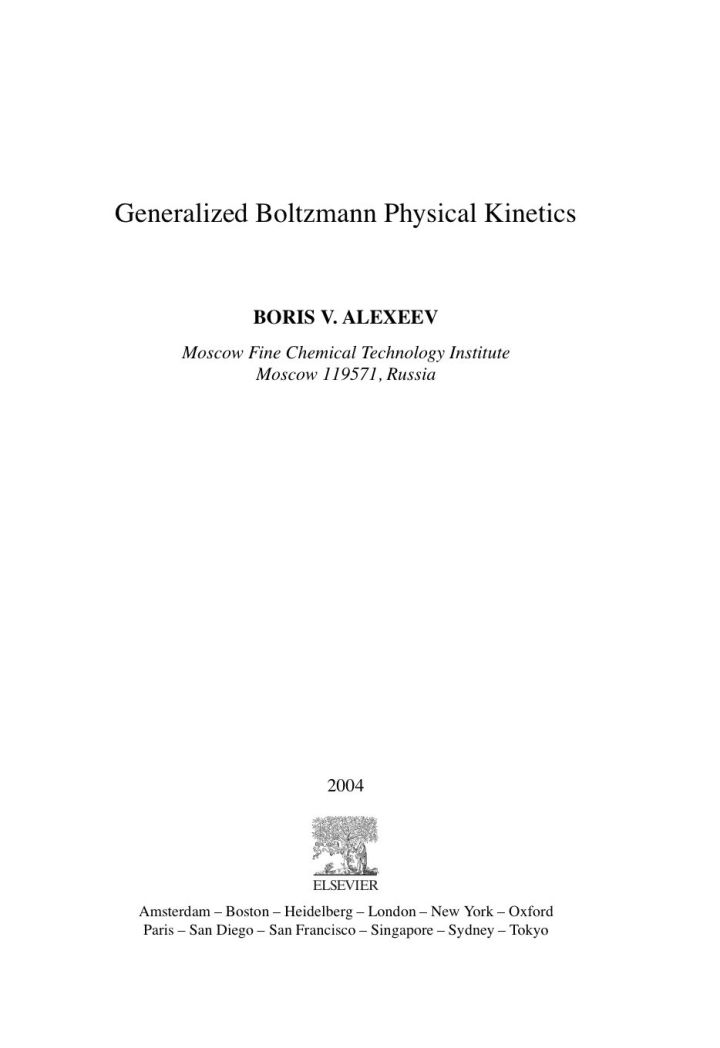# Generalized Boltzmann Physical Kinetics

## Generalized Boltzmann Physical Kinetics

By Alexeev, Boris V.

### Rent or Buy eTextbook

Expires on Apr 18th, 2022
\$210

Publisher List Price: \$214.99
Savings: \$4.99

The most important result obtained by Prof. B. Alexeev and reflected in the book is connected with new theory of transport processes in gases, plasma and liquids. It was shown by Prof. B. Alexeev that well-known Boltzmann equation, which is the basement of the classical kinetic theory, is wrong in the definite sense. Namely in the Boltzmann equation should be introduced the additional terms which generally speaking are of the same order of value as classical ones. It leads to dramatic changing in transport theory. The coincidence of experimental and theoretical data became much better. Particularly it leads to the strict theory of turbulence and possibility to calculate the turbulent flows from the first principles of physics.

· Boltzmann equation (BE) is valid only for particles, which can be considered as material points, generalized Boltzmann equation (GBE) removes this restriction.
· GBE contains additional terms in comparison with BE, which cannot be omitted
· GBE leads to strict theory of turbulence
· GBE gives all micro-scale turbulent fluctuations in tabulated closed analytical form for all flows
· GBE leads to generalization of electro-dynamic Maxwell equations
· GBE gives new generalized hydrodynamic equations (GHE) more effective than classic Navier-Stokes equations
· GBE can be applied for description of flows for intermediate diapason of Knudsen numbers
· Asymptotical solutions of GBE remove contradictions in the theory of Landau damping in plasma

Subject: Mathematics & Statistics -> Mathematics -> Mathematics GeneralPublisher: Elsevier S & T 05/2004
Imprint: Elsevier Science
Language: English
Length: 376 pages

ISBN 10: 0444515828
ISBN 13: 9780444515827
Print ISBN: 9780444515827

Live Chats